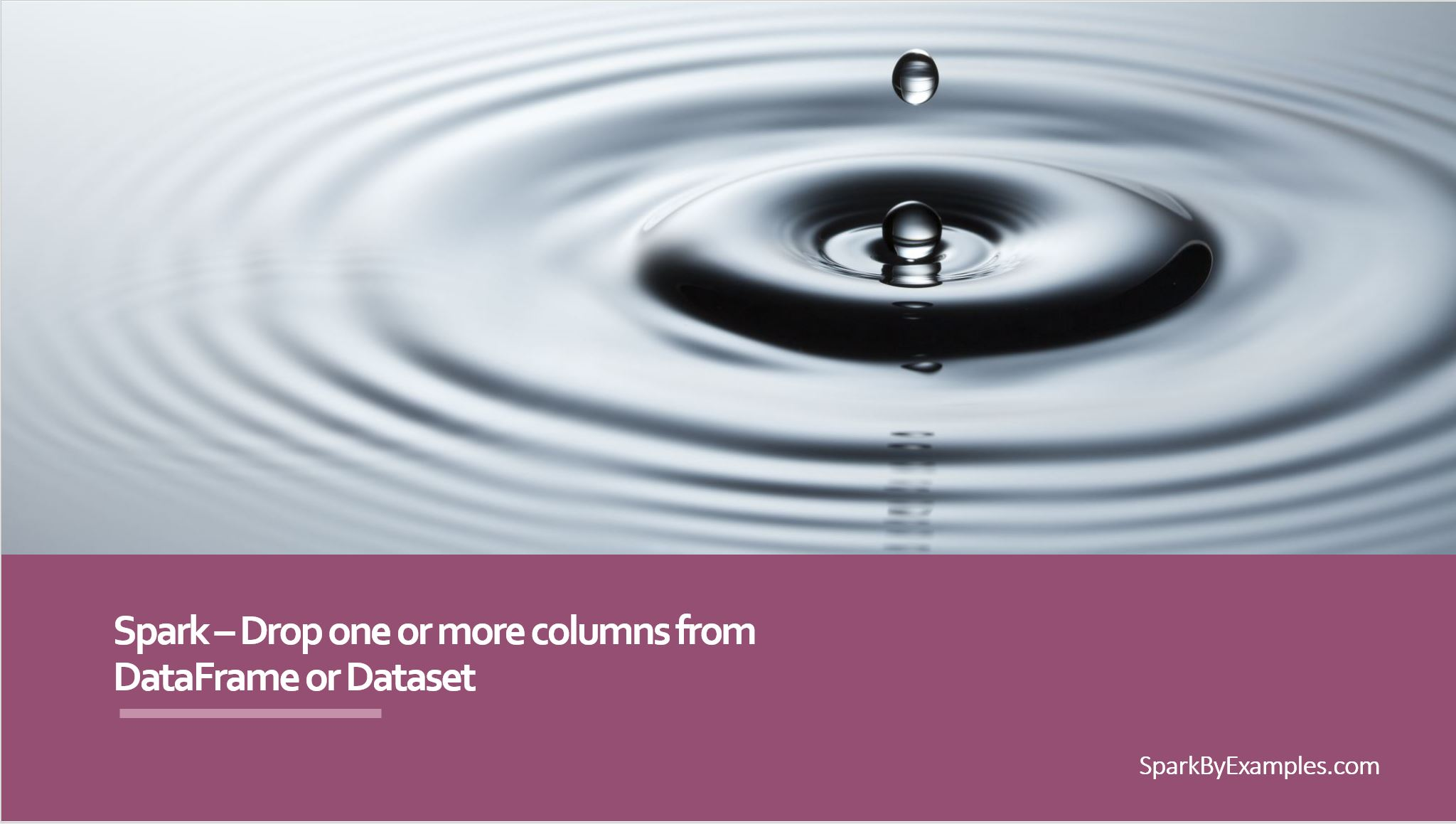# Spark – How to Drop a DataFrame/Dataset column

Spark DataFrame provides a `drop()` method to drop a column/field from a DataFrame/Dataset. drop() method also used to remove multiple columns at a time from a Spark DataFrame/Dataset. In this article, I will explain ways to drop a columns using Scala example.

First, let’s create a DataFrame.

``````
val structureData = Seq(
Row("James","","Smith","36636","NewYork",3100),
Row("Michael","Rose","","40288","California",4300),
Row("Robert","","Williams","42114","Florida",1400),
Row("Maria","Anne","Jones","39192","Florida",5500),
Row("Jen","Mary","Brown","34561","NewYork",3000)
)

val structureSchema = new StructType()

val df = spark.createDataFrame(
spark.sparkContext.parallelize(structureData),structureSchema)
df.printSchema()
``````

This yields below output.

``````
root
|-- firstname: string (nullable = true)
|-- middlename: string (nullable = true)
|-- lastname: string (nullable = true)
|-- id: string (nullable = true)
|-- location: string (nullable = true)
|-- salary: integer (nullable = true)
``````

## Spark DataFrame drop() syntax

Spark `drop()` has 3 different signatures. In the below sections, I’ve explained using all these signatures with examples.

``````
1) drop(colName : scala.Predef.String) : org.apache.spark.sql.DataFrame
2) drop(colNames : scala.Predef.String*) : org.apache.spark.sql.DataFrame
3) drop(col : org.apache.spark.sql.Column) : org.apache.spark.sql.DataFrame
``````

## Drop one column from DataFrame

First and Third signature takes column name as String type and Column type respectively. When you use the third signature make sure you import `org.apache.spark.sql.functions.col`

``````
val df2 = df.drop("firstname") //First signature
df2.printSchema()

df.drop(df("firstname")).printSchema()

//import org.apache.spark.sql.functions.col is required
df.drop(col("firstname")).printSchema() //Third signature
``````

The above 3 examples drops column “firstname” from DataFrame. You can use either one of these according to your need.

``````
root
|-- middlename: string (nullable = true)
|-- lastname: string (nullable = true)
|-- id: string (nullable = true)
|-- location: string (nullable = true)
|-- salary: integer (nullable = true)
``````

## Drop multiple columns from DataFrame

This uses second signature of the drop() which removes more than one column from a DataFrame.

``````
//Refering more than one column
df.drop("firstname","middlename","lastname")
.printSchema()

// using array/sequence of columns
val cols = Seq("firstname","middlename","lastname")
df.drop(cols:_*)
.printSchema()
``````

The above two examples remove more than one column at a time from DataFrame. These both yield the same output.

``````
root
|-- id: string (nullable = true)
|-- location: string (nullable = true)
|-- salary: integer (nullable = true)
``````

## Complete Example

Below is a complete example of how to drop one column or multiple columns from a Spark DataFrame.

``````
package com.sparkbyexamples.spark.dataframe.examples

import org.apache.spark.sql.{Row, SparkSession}
import org.apache.spark.sql.types.{IntegerType, StringType, StructType}
import org.apache.spark.sql.functions.col
object DropColumn extends App {

val spark:SparkSession = SparkSession.builder()
.master("local")
.appName("SparkByExamples.com")
.getOrCreate()

val data = Seq(
Row("James","","Smith","36636","NewYork",3100),
Row("Michael","Rose","","40288","California",4300),
Row("Robert","","Williams","42114","Florida",1400),
Row("Maria","Anne","Jones","39192","Florida",5500),
Row("Jen","Mary","Brown","34561","NewYork",3000)
)

val schema = new StructType()

val df = spark.createDataFrame(
spark.sparkContext.parallelize(data),schema)
df.printSchema()
df.show(false)

df.drop(df("firstname"))
.printSchema()

df.drop(col("firstname"))
.printSchema()

val df2 = df.drop("firstname")
df2.printSchema()

df.drop("firstname","middlename","lastname")
.printSchema()

val cols = Seq("firstname","middlename","lastname")
df.drop(cols:_*)
.printSchema()
}

``````

This complete example is also available at Spark Examples Github project for references.

Thanks for reading and Happy Learning !!

## Related Articles

### NNK

SparkByExamples.com is a Big Data and Spark examples community page, all examples are simple and easy to understand and well tested in our development environment Read more ..

### This Post Has 3 Comments

1.Anonymous

Hi NNK,

Thanks for sharing such informative knowledge.
Can you also share how to write CSV file faster using spark scala.

Thanks

2.Maheswaran

Hi nnk, all your articles are really awesome. I want to debug spark application. Tools I m using are eclipse for development, scala, spark, hive. Can you post something related to this. Thank you.

1.NNK

Thanks for your kind words. Sure will do an article on Spark debug.